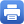Columbia Home
What PhD Graduate Students are Assumed to Know

Below are listed basic topics from various areas of mathematics. The first-year graduate courses in the Department are predicated on the assumption that all entering Ph.D. students are familiar with all these topics. As a practical matter, the Department knows that this will not be the case – every student will have gaps in his or her background. The Department strongly encourages every entering student, in the summer before his or her first year, to go over these topics, using the listed references or other equivalent books, and review (or learn) this material.

Linear Algebra: Finite dimensional vector spaces (over R) and linear maps between them – subspaces, quotient spaces, dimension, bases, matrix representations. Positive definite inner products, orthonormal bases, extensions of orthonormal subsets. Eigenvalues and eigenvectors for automorphisms. Characteristic polynomial.

References: M. Artin, ‘Algebra‘ (Prentice Hall, 1991), Chapters 1,3,4
K. Hoffman and R. Kunze, ‘Linear Algebra‘, Chapters 1-6, (Prentice-Hall, 1971).

Abstract Algebra: Definitions of groups, rings, fields, and modules over a ring. Homomorphisms of these objects. Subgroups, normal subgroups, quotient groups. Cyclic groups, finite abelian groups (structure theorem). Ideals, prime and maximal and their quotients — basic examples Z, k[X], rings of algebraic integers. Field extensions, splitting fields of polynomials, normal extensions.

References: M. Artin, ‘Algebra‘, Chapters 2, 10, 11, 12, 13, 14
I. Herstein, ‘Topics in Algebra‘ (Blaisdell Publishers, 1964).

Point-set Topology
: Open and closed sets, continuous functions. Connectedness, compactness, Hausdorff, normality. Metric spaces, Rn. Heine-Borel theorem.

Reference: J. Munkres, ‘Topology, A First Course‘, Part I (Prentice-Hall).

Calculus: Differential of a smoothing mapping between open subsets in Euclidean spaces. Matrix of partial derivatives. Inverse and implicit functions. Multivariable Riemann integration.

References: W. Rudin, ‘Principles of Mathematical Analysis‘ (McGraw-Hill, 1964)
A. Browder, ‘Mathematical Analysis: An Introduction‘ (Springer, 1996).

Complex Analysis
: Definition of holomorphic functions, Cauchy integral formula, power series representations of holomorphic functions, radius of convergence, meromorphic functions, residues.

Reference: L. Ahlfors, ‘Complex Analysis‘, (McGraw-Hill, 1973), Chapters 1- 5.

Real Analysis
: A thorough working knowledge of advanced calculus, at the level of the books of W. Rudin or A. Browder as listed under Calculus. Additional topics: Pointwise uniform convergence of functions, equi-continuity, l2, L2(S1), Hilbert spaces, orthonormal bases.

### Other Introductory Material

For those entering students whose backgrounds are strong and who wish to do reading before entering graduate school to enrich their knowledge of mathematics the Department suggests the following books. The Department considers each of these to be a well-written introduction which conveys the flavor of the subject. Looking at these books may help give a feeling for various subspecialities within mathematics.

• L. Ahlfors, ‘Complex Analysis‘ (McGraw-Hill, 1973).
• V. Arnold, ‘Ordinary Differential Equations‘ (MIT Press)
• M. Artin, ‘Algebra‘ (Prentice Hall, 1991)
• F. Kirwan, ‘Complex Algebraic Curves‘, London Mathematical Series Student Texts 23 (Cambridge, 1992).
• W. Massey, ‘A Basic Course in Algebraic Topology‘ Graduate Texts in Mathematics Vol. 127 (Springer-Verlag, 1991).
• J. Milnor, ‘Topology from the Differentiable Viewpoint
• S. Ross, ‘A First Course in Probability‘ (5th edition, Prentice-Hall, 1997).
• W. Rudin, ‘Real and Complex Analysis
• J-P. Serre, ‘A course in Arithmetic
• J-P. Serre, ‘Linear Representations of Finite GroupsPrint this page